## Deep Learning with Keras and TensorFlow

Certification Training
7576 Learners
7 Chapters +

# Deep Learning Tutorial

This is the introductory lesson of the Deep Learning tutorial, which is part of the Deep Learning Certification Course (with TensorFlow). In this lesson, we will be introduced to Deep Learning, its purpose, and the learning outcomes ofthe tutorial.

Let us look at the objectives of this lesson.

## Objectives

By the end of this Deep Learning Tutorial, you will be able to:

• Gain in-depth knowledge of TensorFlow along with its functions, operations, and the execution pipeline

• Implement linear regression and gradient descent in TensorFlow

• Understand the concept of artificial neural networks, convolutional neural networks, and recurrent neural networks

• Discuss how to speed up neural networks along with regularization techniques to reduce overfitting

• Implement deep learning algorithms, and learn how to train deep networks

• Understand the use cases of implementing artificial intelligence such as image processing, natural language processing, speech recognition, deep face - facial recognition system, etc.

Let us begin by introducing Machine Learning in the next section.

## What is Machine Learning?

Artificial Intelligence (AI) systems learn by extracting patterns from input and output data.

Machine Learning (ML) relies on learning patterns based on sample data. Programs learn from labeled data (supervised learning), unlabelled data (unsupervised learning), or a combination of both (semi-supervised learning).

Artificial Intelligence (AI) came around in the middle of 1900s when scientists tried to envision intelligent machines. Machine Learning evolved in the late 1900s. This allowed scientists to train machines for AI.

In the early 2000s, certain breakthroughs in multi-layered neural networks facilitated the advent of Deep Learning.

You too can join the high earners’ club. Enroll in our Deep Learning Course and earn more today.

## What is Deep Learning?

Deep Learning is a specialized form of Machine Learning that uses supervised, unsupervised, or semi-supervised learning to learn from data representations.

It is similar to the structure and function of the human nervous system, where a complex network of interconnected computation units work in a coordinated fashion to process complex information.

Machine Learning is an approach or subset of Artificial Intelligence that is based on the idea that machines can be given access to data along with the ability to learn from it. Deep Learning takes Machine Learning to the next level.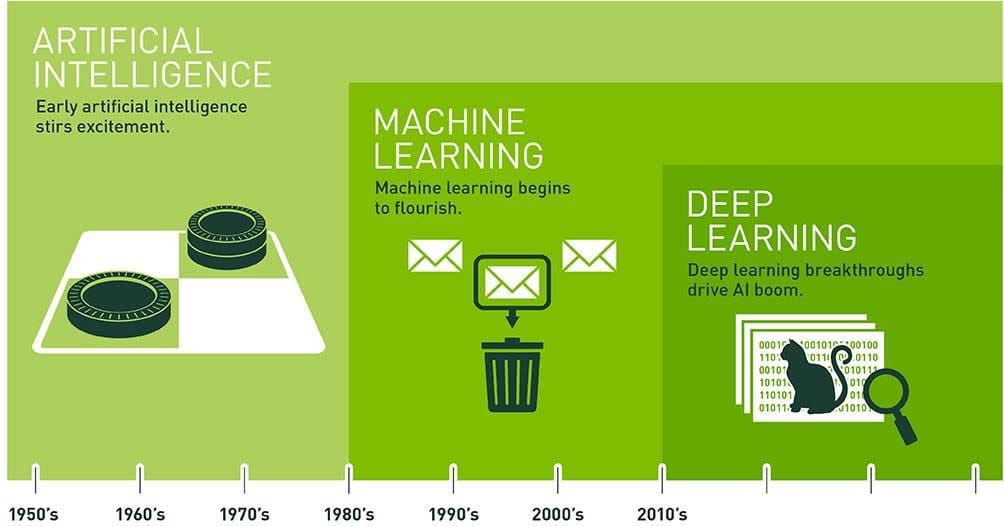There are many aspects of Deep Learning as listed below. These will be covered in detail in the subsequent chapters.

1. Multiple levels of hierarchical representations

2. Multi-layered neural networks

3. Training of large neural networks

4. Multiple non-linear transformations

5. Pattern recognition

6. Feature extraction

7. High-level data abstractions model

In the next section, let us discuss the relationship between Artificial Intelligence and Deep Learning.

## Relationship Between Artificial Intelligence And Deep Learning

Machine Learning is an approach or subset of Artificial Intelligence that is based on the idea that machines can be given access to data along with the ability to learn from it. Deep Learning takes Machine Learning to the next level.

Let us discuss traditional to deep learning at a glance in the next section.

## Traditional to Deep Learning at a Glance

As you go from the rule-based systems to the deep learning ones, more complex features and input-output relationships become learnable.

In the next section, let us discuss the drivers of Deep Learning.

## Drivers of Deep Learning

The drivers of Deep Learning are listed below:

• Availability of multi-layered learning network

• Ability to leverage Big Data

• Expanded use of high-performing graphics processing units (GPU)

• An improved scale of data and size of neural networks

• Improved performance of Neural Networks

• Availability of a large amount of labeled data

• The ability of GPUs to perform parallel computing

Let us next look into a case study of Sowing App which is based on Deep Learning.

## Case Study: Sowing App

The timing of sowing is the biggest differentiator between a good and a failed crop, especially for rainfed crops.

Microsoft India collaborated with ICRISAT (International Crop Research Institute for the Semi-Arid Tropics) to develop a “Sowing App.” The app guides farmers on soil conditions and weather and provides rainfall predictions. It helps them get a higher crop yield and have better price control.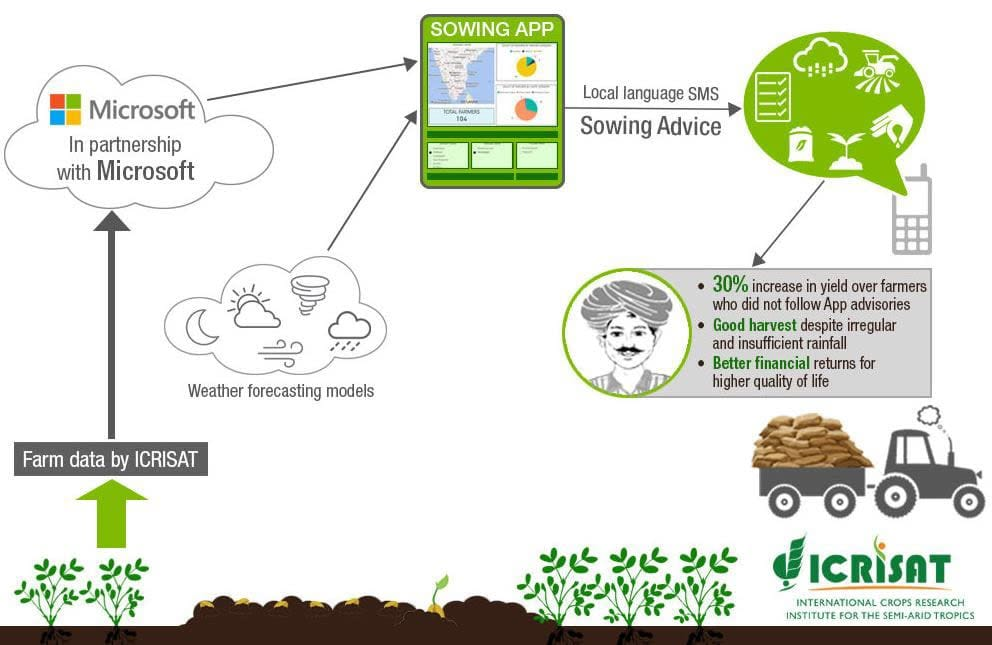The app calculates crop yield using data from geostationary satellite images. Notifications are sent to farmers on their phones in their native language such as:

• Short term weather prediction, especially rainfall

• Soil quality data

• Previous crop history

• When to sow

• When not to sow

• When soil moisture is sufficient for seed germination

• Pest threats to the crops

• Price forecasts for the crops

The pilot was implemented in Devanakonda Mandal in Kurnool district of Andhra Pradesh for the groundnut crop. The Sowing advisory was developed in 2016 and used by 175 farmers in a pilot phase. The app is now being scaled in all 13 districts. Farmers in a few villages in the following states are now using this app:

• Karnataka

• Maharashtra

• Andhra Pradesh

• Madhya Pradesh

• Telangana

Azure cloud platform was used to deploy this app, along with Artificial Intelligence, Machine Learning, Big Data, and Analytics. The technologies that are used to power the app are:

• Cloud Machine Learning

• Satellite imagery

• Advanced analytics

• Microsoft Cortana Intelligence Suite

• Machine Learning

• Power BI

Climate data for the Devanakonda area of Andhra Pradesh from 1986 to 2015 was collected to predict the crop sowing period.

In the next section, let us discuss the path of Deep Learning.

## Deep Learning Path

The path to master Deep Learning can be divided into four phases:

1. Introduction - You begin with an introduction to the idea of Deep Learning

2. Applied Math and Machine Learning Basics - Review the basics of Math and core Machine Learning algorithms used later in Deep Learning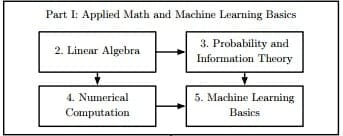1. Deep Networks - Learn the most popular forms of Deep Learning neural networks prevalent currently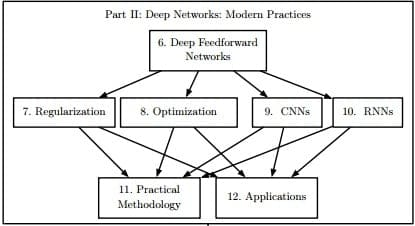1. Deep Learning Research - Finally review some of the more recent advances in Deep LearningIn the next section, let us learn the Artificial Neural Networks.

Preparing for a career in Deep Learning? Check out our Course Preview on Deep Learning here!

## Artificial Neural Networks

In the coming sections, we would learn about Artificial Neural Networks starting with Biological Neuron.

### Biological Neuron

A mammalian brain has billions of neurons. Neurons are interconnected nerve cells in the human brain that are involved in processing and transmitting chemical and electrical signals. They take input and pass along outputs.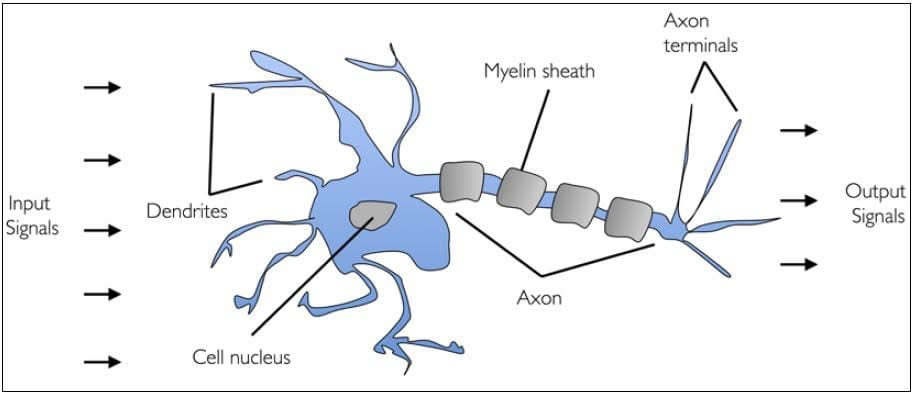A human brain can learn how to identify objects from photos. For example, it can learn to identify the characteristics of chairs and thereby increase its probability of identifying them over time.

Following are the important parts of the biological neuron:

• Dendrites - branches that receive information from other neurons

• Cell nucleus or Soma - processes the information received from dendrites

• Axon - a cable that is used by neurons to send information

• Synapse - the connection between an axon and other neuron dendrites

## Human Brain vs. Artificial Neural Networks

The computational models in Deep Learning are loosely inspired by the human brain. The multiple layers of training are called Artificial Neural Networks (ANN).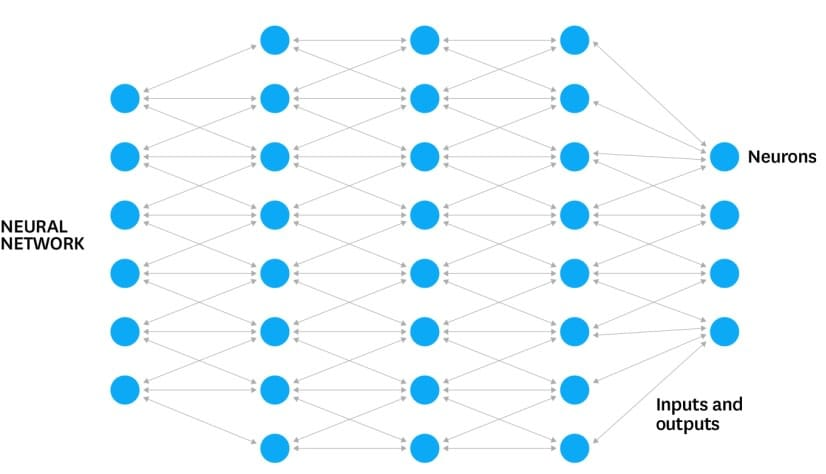ANNs are processing devices (algorithms or actual hardware) that are modeled on the neuronal structure of the mammalian cerebral cortex but on a much smaller scale. It is a computing system made up of a number of simple, highly interconnected processing elements which process information through their dynamic state response to external inputs.

## Artificial Neural Networks Process

Artificial Neural Networks consist of the following four main parts:

• Neurons

• Nodes

• Input

• Output

Let us discuss each of them in detail.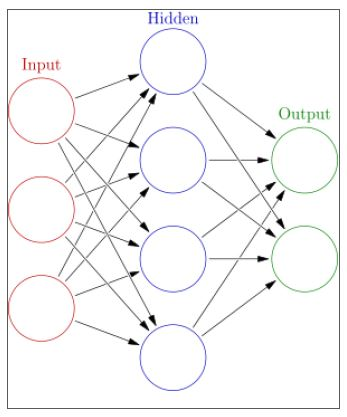Neuron

Artificial Neural Networks contain layers of neurons. A neuron is a computational unit that calculates a piece of information based on weighted input parameters. Inputs accepted by the neuron are separately weighted.

Inputs are summed and passed through a non-linear function to produce output. Each layer of neurons detects some additional information, such as edges of things in a picture or tumors in a human body. Multiple layers of neurons can be used to detect additional information about input parameters.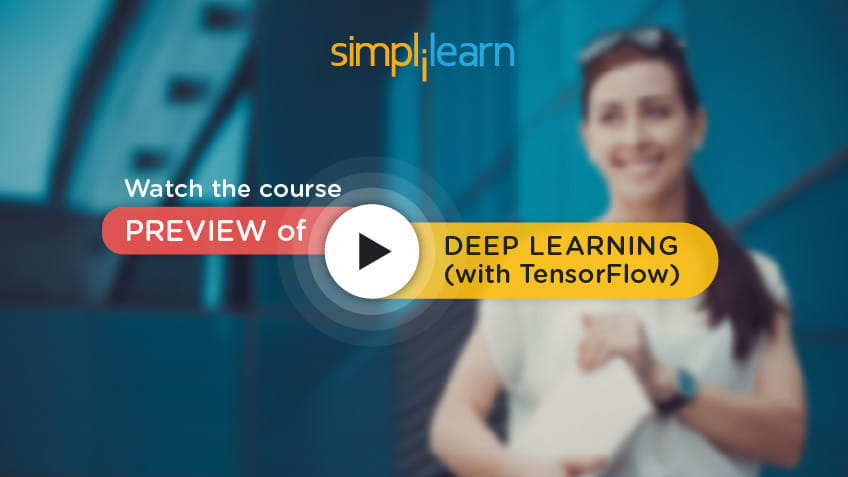Nodes

Artificial Neural Network is an interconnected group of nodes akin to the vast network of layers of neurons in a brain. Each circular node represents an artificial neuron and an arrow represents a connection from the output of one neuron to the input of another.

Inputs

Inputs are passed into the first layer. Individual neurons receive the inputs, with each of them receiving a specific value. After this, an output is produced based on these values.

Outputs

The outputs from the first layer are then passed into the second layer to be processed. This continues until the final output is produced. The assumption is that the correct output is predefined.

Each time data is passed through the network, the end result is compared with the correct one, and tweaks are made to their values until the network creates the correct final output each time.

In the next section, let us study the types of Neural Networks.

## Types of Neural Networks

Some of the commonly used neural networks are as follows:

• Artificial Neural Network (ANN)

• Convolutional Neural Network (CNN)

• Recurrent Neural Network (RNN)

• Deep Neural Network (DNN)

• Deep  Belief Network (DBN)

The use cases of different neural networks are listed below:

 Neural Network Use Case ANN Computational Neuroscience CNN Image Processing RNN Speech Recognition DNN Acoustic Modeling DBN Drug Discovery

Next, let us understand what Deep Face is in the following case study.

## Case Study: Deep Face

DeepFace is Facebook’s facial recognition system created using Deep Learning. It uses a nine-layer neural network with more than 120 million connection weights. The researchers used four million images uploaded by Facebook users. Facebook has been using this technology since 2015.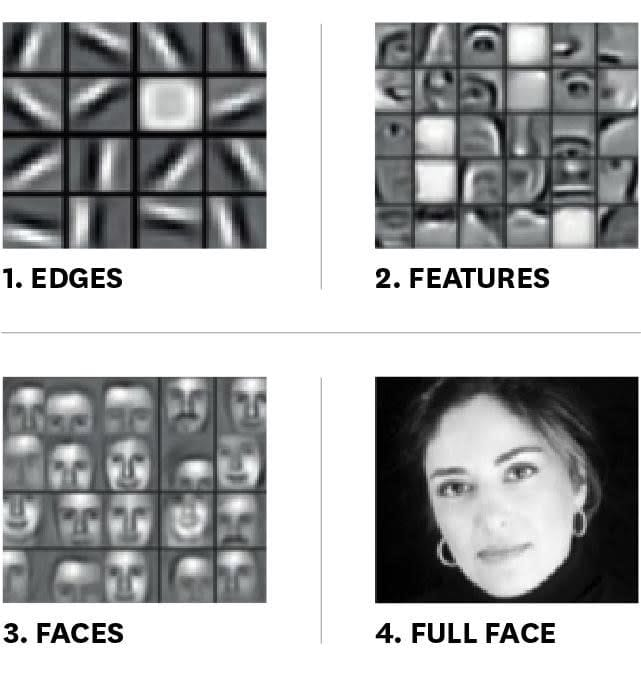Layers of virtual neurons are trained to identify edges, features, a face, and so on. In the example given below, the first layer recognizes edges.

The second layer recognizes facial features like a nose or an ear. The third layer recognizes faces. The full face is eventually recognized in the fourth layer.

The layers of a neural network work in coordination with each other to progressively add more insight as the data passes from input layers toward the output layers.

In the next section, let us look at the Deep Learning Platforms.

## Deep Learning Platforms

The CPU and GPU Specifications are discussed through the table below:

 Specifications Intel Core i7-6900k Processor Extreme Ed. NVIDIA GeForce GTX I 080 Ti Base Clock Frequency 3.2 GHz < 1.5 GHz Cores 8 3584 Memory Bandwidth 64 GB/s 484 GB/s Floating-Point Calculations 409 GFLOPS 11300 GFLOPS Cost ~\$1000.00 ~\$700.00

The table shows that GPUs (right column) are cheaper than modern CPUs (middle column) for deep learning tasks. In addition, they support a lot more cores and calculations.

Python Specifications

Python is limited to execution on one core due to the Global Interpreter Lock (GIL). Multiprocessing library in Python allows distributed computation over several cores, but most advanced desktop hardware comes only with a maximum of 8 to 16 cores.

GPU: Special Packages

In image processing, there can be an explosion in the number of parameters. Single processor units cannot handle these easily, but GPUs can. Each GPU is akin to a small computer cluster. However, one has to use special packages like CUDA or OpenCL to write code for GPUs.

Deep Learning library like TensorFlow makes it easy to write code for either OpenCL or CUDA-enabled GPUs.

Deep Learning platforms include:

• Tensorflow(Python Based)

• Keras(Python)

• Torch( C/C++)

• Deeplearning4j(JAVA)

In the next section, let us see what are the benefits of Deep Learning Tutorial to professionals.

## Benefits of Deep Learning Tutorial to Professionals

With the advancement in technology, there has been a considerable rise in demand for engineers who are proficient in deep learning. This deep learning tutorial is ideal for the professionals listed below:

• Software engineers

• Data scientists

• Data analysts

• Statisticians with interest in deep learning

Let us look at the prerequisites for Deep Learning in the next section.

Wish to have in-depth knowledge of Deep Learning? Consider watching our Course Preview now!

## Prerequisites for Deep Learning

Candidates willing to pursue this Deep Learning Tutorial should have:

• An understanding of the fundamentals of Python programming

• A basic knowledge of statistics

• A basic machine learning knowledge

Next, let us focus on the lessons covered in this Deep Learning Tutorial.

## Lessons Covered in the Deep Learning Tutorial

This Deep Learning tutorial covers a total of 9 chapters. Given below is a small description of all the chapters.

 Lesson No Chapter Name What You’ll Learn Lesson 1 Introduction to Deep Learning In this chapter, you’ll be able to: Understand the evolution of Deep Learning from Artificial Intelligence and Machine Learning Describe the meaning and definition of Deep Learning with the help of a case study Explore the meaning, process, and types of neural networks with a comparison to human neurons Identify the platforms and programming stacks used in Deep Learning Lesson 2 Perceptron In this chapter, you’ll be able to: Explain artificial neurons with a comparison to biological neurons Implement logic gates with Perceptron Discuss Sigmoid units and Sigmoid activation function in Neural Network Describe ReLU and Softmax Activation Functions Explain Hyperbolic Tangent Activation Function Lesson 3 How to train an Artificial Neural Network In this chapter, you’ll be able to: Understand how ANN is trained using Perceptron learning rule. Explain the implementation of Adaline rule in training ANN. Describe the process of minimizing cost functions using Gradient Descent rule. Analyze how learning rate is tuned to converge an ANN. Explore the layers of an Artificial Neural Network(ANN). Lesson 4 Multilayer ANN In this chapter, you’ll be able to: Analyze how to regularize and minimize the cost function in a neural network Carry out backpropagation to adjust weights in a neural network Inspect convergence in a multilayer ANN Explore multilayer ANN Implement forward propagation in multilayer perceptron (MLP) Understand how the capacity of a model is affected by underfitting and overfitting Lesson 5 Introduction to TensorFlow In this chapter, you’ll be able to: Explore the meaning of TensorFlow Create a computational and default graph in TensorFlow Demonstrate reshaping of a tensor with tf.reshape Implement Linear Regression and Gradient Descent in TensorFlow Discuss the meaning and application of Layers and Keras in TensorFlow Demonstrate the use of TensorBoard Lesson 6 Training Deep Neural Nets In this chapter, you’ll be able to: Discuss solutions to speed up neural networks Explain regularization techniques to reduce overfitting Lesson 7 Convolutional Neural Networks In this chapter, you’ll be able to: Learn how to implement CNNs within TensorFlow Discuss the process of convolution and how it works for image processing or other tasks Describe what CNNs are and their applications Illustrate how zero padding works with variations in kernel weights Elaborate the pooling concepts in CNNs Lesson 8 Recurrent Neural Networks In this chapter, you’ll be able to: Explore the meaning of Recurrent Neural Networks (RNN) Understand the working of recurrent neurons and their layers Interpret how memory cells of recurrent neurons interact Implement RNN in TensorFlow Demonstrate variable length input and output sequences Lesson 9 Other forms of Deep Learning In this chapter, you’ll be able to: Elaborate on the functionality of an autoencoder and its various types Discuss the working and uses of reinforcement learning Describe the working of Generative-Adversarial Networks

## Summary

Let us summarize what we have learned in this lesson:

• Deep Learning is a subset of Machine Learning and Artificial Intelligence and makes complex features and input-output relationships learnable.

• New breakthroughs in neural networks, availability of Big Data, and low-cost high-performance GPU chips are driving the Deep Learning revolution.

• Deep Learning is useful for complex intelligence tasks like face recognition, speech recognition, machine translation etc.

• Artificial Neural Network is a computing system made up of a number of simple, highly interconnected processing elements that process information through their dynamic state response to external inputs. It is modeled on the inter-connected neurons in the human brain.

• Each circular node represents an artificial neuron, and an arrow represents a connection between the output of one neuron to the input of another.

• Some of the commonly used neural networks are RNN, CNN, ANN, DNN, and DBN.

• GPUs are cheaper than modern CPUs now, in addition to supporting a lot more cores and calculations.

## Conclusion

With this, we come to an end about what this introductory lesson on Deep Learning tutorial includes. In the next chapter, we will discuss the Perceptron.

Related Courses
Learner Reviews
Related Articles
• Disclaimer
• PMP, PMI, PMBOK, CAPM, PgMP, PfMP, ACP, PBA, RMP, SP, and OPM3 are registered marks of the Project Management Institute, Inc.

#### Request more information

###### For business
Name*
Email*
Phone Number*
Your Message (Optional)
###### Our consultants will get in touch with you soon.

A Simplilearn representative will get back to you in one business day.

First Name*
Last Name*
Work Email*
Phone Number*
Company*
Job Title*